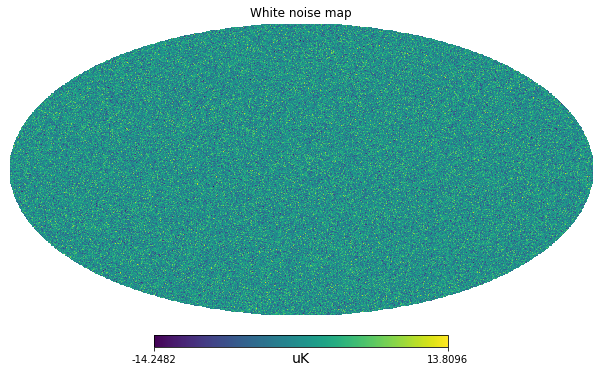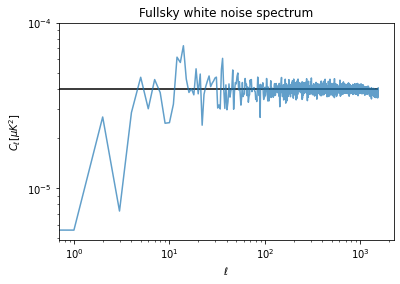# Handle white noise with healpy 1 Full sky coverage

Simulate white noise maps and use hitmaps
cosmology
python
healpy
Published

June 19, 2020

import healpy as hp
import numpy as np
%matplotlib inline
import matplotlib.pyplot as plt
import astropy.units as u

In this series of notebooks, we will understand how to handle white noise in the case of an experiment with sky observations which are both not-uniform and have partial sky coverage.

Let’s first start assuming a sensitivity of an experiment array of detectors of $$10 \mu K \sqrt(s)$$.

# Number based on Simons Observatory SAT UHF1 array of detectors

net = 10. * u.Unit("uK * sqrt(s)")

5 years with a efficiency of 20%:

integration_time_total = 5 * u.year * .2

## Uniform full sky survey

As a reference, let’s first start with the trivial case of uniform full sky coverage, i.e. we spend the same amount of observation time in each pixel.

nside = 512
npix = hp.nside2npix(nside)
standard_deviation_per_pixel = (net / np.sqrt(integration_time_total/npix)).decompose()
standard_deviation_per_pixel

$$3.1572473 \times 10^{-6} \; \mathrm{K}$$

m = np.random.normal(scale = standard_deviation_per_pixel.value, size=npix) * standard_deviation_per_pixel.unit
m = m.to(u.uK)
hp.mollview(m, unit=m.unit, title="White noise map")## Power spectrum

Finally we can compute the angular power spectrum with anafast, i.e. the power as a function of the angular scales, from low $$\ell$$ values for large angular scales, to high $$\ell$$ values for small angular scales.

At low $$\ell$$ there is not much statistics and the power spectrum is biased, but if we exclude lower ells, we can have an estimate of the white noise $$C_\ell$$ coefficients. We can then compare with the theoretical power computed as:

$C_\ell = \Omega_{pix}\sigma^2$

Where: $$\Omega_{pix}$$ is the pixel are in square-radians and $$\sigma^2$$ is the white noise variance.

cl = hp.anafast(m)
cl[100:].mean()
3.9283892627207396e-05
m.std()

$$3.1567443 \; \mathrm{\mu K}$$

pixel_area = hp.nside2pixarea(nside)
white_noise_cl = (standard_deviation_per_pixel**2 * pixel_area).to(u.uK**2)
white_noise_cl

$$3.9820426 \times 10^{-5} \; \mathrm{\mu K^{2}}$$

plt.figure(figsize=(6,4))
plt.loglog(cl, label="Map power spectrum", alpha=.7)
plt.hlines(white_noise_cl.value, 0, len(cl), label="White noise level")
plt.title("Fullsky white noise spectrum")
plt.xlabel("$\ell$")
plt.ylabel("$C_\ell [\mu K ^ 2]$");